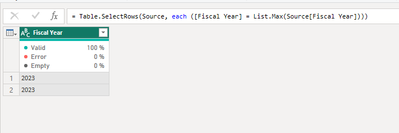cancel
Showing results for
Did you mean:Helper III

## How to filter by the maximum value in a field?

Hello,

Say I had a table of transactions, and there was a field with fiscal year. Please can you tell me how to only return records who's fiscal year is equal to the maximum in that column (i.e. the latest fiscal year)

Thank you,

PW

1 ACCEPTED SOLUTIONSolution Sage

Hi @PowerWhy ,

A possible solution:``````let
Source = Table.FromRows(Json.Document(Binary.Decompress(Binary.FromText("i45WMjIwMlCK1UFlGMIYRjCGMRIjFgA=", BinaryEncoding.Base64), Compression.Deflate)), let _t = ((type nullable text) meta [Serialized.Text = true]) in type table [#"Fiscal Year" = _t]),
#"Filtered Rows" = Table.SelectRows(Source, each ([Fiscal Year] = List.Max(Source[Fiscal Year])))
in
#"Filtered Rows"``````

If this post answered your question, please mark it as a solution to help other users find useful content.
Kudos are another nice way to acknowledge those who tried to help you.

J. Payeras
Mallorca, Spain
2 REPLIES 2Community Support

Hi @PowerWhy ,

You could try the Group By in Power Query.

Here're the steps.

1.Load the table of transactions into Power Query.

2.Group the table by the fiscal year field.

3.Add an aggregation step to find the maximum value of the fiscal year field.

4.Merge the maximum value back into the original table using a left outer join.

5.Filter the table to only include rows where the fiscal year field is equal to the maximum value.

Best Regards,

Stephen Tao

If this post helps, then please consider Accept it as the solution to help the other members find it more quickly.Solution Sage

Hi @PowerWhy ,

A possible solution:``````let
Source = Table.FromRows(Json.Document(Binary.Decompress(Binary.FromText("i45WMjIwMlCK1UFlGMIYRjCGMRIjFgA=", BinaryEncoding.Base64), Compression.Deflate)), let _t = ((type nullable text) meta [Serialized.Text = true]) in type table [#"Fiscal Year" = _t]),
#"Filtered Rows" = Table.SelectRows(Source, each ([Fiscal Year] = List.Max(Source[Fiscal Year])))
in
#"Filtered Rows"``````

If this post answered your question, please mark it as a solution to help other users find useful content.
Kudos are another nice way to acknowledge those who tried to help you.

J. Payeras
Mallorca, Spain